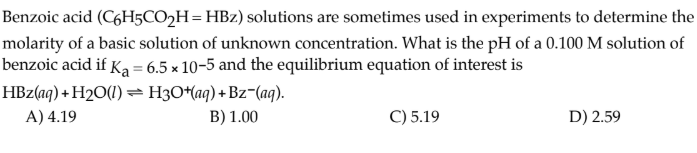# Benzoic acid (C6H5CO2H = HBz) solutions are sometimes used in experiments to determine the molarity of a basic solution of unknown concentration. What is the pH of a 0.100 M solution of benzoic acid if Ka = 6.5 × 10^-5 and the equilibrium equation of interest is HBz(aq) + H2O(l) ⇌ H3O+(aq) +Bz-(aq). A) 4.19 B) 1.00 C) 5.19 D) 2.59